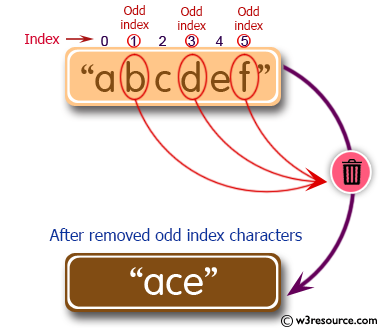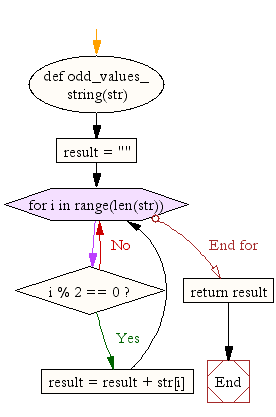﻿ Python: Remove the characters which have odd index values of a given string - w3resource

# Python: Remove the characters which have odd index values of a given string

## Python String: Exercise-11 with Solution

Write a Python program to remove characters that have odd index values in a given string.Sample Solution:-

Python Code:

``````def odd_values_string(str):
result = ""
for i in range(len(str)):
if i % 2 == 0:
result = result + str[i]
return result

print(odd_values_string('abcdef'))
print(odd_values_string('python'))
```
```

Sample Output:

```ace
pto
```

Flowchart:## Visualize Python code execution:

The following tool visualize what the computer is doing step-by-step as it executes the said program:

Python Code Editor:

Have another way to solve this solution? Contribute your code (and comments) through Disqus.

What is the difficulty level of this exercise?

Test your Programming skills with w3resource's quiz.

﻿

## Python: Tips of the Day

Decapitalizes the first letter of a string:

Example:

```def tips_decapitalize(s, upper_rest=False):
return s[:1].lower() + (s[1:].upper() if upper_rest else s[1:])
print(tips_decapitalize('PythonTips'))
print(tips_decapitalize('PythonTips', True))
```

Output:

```pythonTips
pYTHONTIPS
```

We are closing our Disqus commenting system for some maintenanace issues. You may write to us at reach[at]yahoo[dot]com or visit us at Facebook